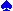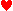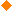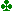# Zar Points by Zar Petkov Part 1

#### ByZar Petkov

Feb 1, 2014#### Source: [button link=»http://www.bridgeguys.com/» size=»small» window=»yes»]BridgeGuys[/button]

Never miss a game again? That’s easy – simply bid a game on every board 🙂 While Bob Hamman’s note that “Bidding is only 3% of the game of bridge” may be true, if you don’t bid your games you certainly cannot catch up by making 13 tricks with brilliant play at your 2contract, while your not-so-brilliant opponents make only 10 tricks at their 4contract. The experts know that, though – and they bid “aggressive” games that “somehow, magically” turn out to be cold. Experts use their expert judgment which advanced and intermediate players just don’t have yet. This article presents the tool for the advanced and intermediate players to get this expert-level “aggressive” judgment and never miss a game again – be it a “somehow-magical” or just a “regular, plain” contract, and to stop at a part-score when no game is in sight.

#### 1) The Opening

The Zar-Points theory is a result of exhaustive research of hundreds and hundreds of “aggressive” game contracts bid by world-class experts like Hamman, Wolff, Meckwell, Lauria, DeFalco, Zia, Helgemo, Chagas, Sabine Auken, Karen McCallum (I have great respect for the women experts) and many others at various world-class tournaments.

Following the 80-20 rule, hand evaluation is 80% Initial evaluation and 20% evaluation adjustment as the bidding progresses. The initial evaluation (just as you pick up your cards and have a look at what’s in there) captures the three standard important aspects of every hand: the shape, the controls, and the standard (Milton Works 4-3-2-1) HCP. The re-evaluation covers the placement of the honors and the suit-lengths in the light of partner’s and opponents’ bidding.

Here is the simple quick description of the initial hand evaluation (Zar Points or Zars).

Calculating the Zar Points has 2 parts – calculating the High-card Points (HP) and the Distribution Points (DP).

For the high-card points we use the 6-4-2-1 scheme which adds the sum of your controls (A=2, K=1) to your standard Milton HCP, in the 4-3-2-1 scheme (A=4, K=3, Q=2, J=1). You will see WHY we have adopted this HP counting in the second part of the article, but the short answer is: NOT because “we feel that this is the best way” 😆

Calculating distribution points is not news in Bridge – Charles Goren introduced the Goren Points more than half-a-century ago. It counts 3 points for every void, 2 points for every singleton, and 1 point for every doubleton. You understand, of course, that indirectly it also holds implicit valuation for the long suits, since the sum of all the 4 lengths is 13 – so, for example, the flat 4-3-3-3 distribution gives you 0 Goren distribution points, while with 5-5 two-suiter you get 3 Goren points (either 2+1 for a singleton and a doubleton or 3 for a void).

As we are going to see, there are only 39 different possible distributions in a bridge hand. To get a feel of the enormous amount of hands these 39 “types” of distribution represent, just asks yourself how many deals are there, in which YOU, sitting in the dealer’s position (East, throughout this article) get a 13-0-0-0 distribution. So – how many do you think?

The answer may surprise you –  337, 912, 392, 291, 465, 600

DIFFERENT deals in which you’ll have 13-0-0-0 distribution – the LEAST probable distribution! How about the MOST probable distribution of 4-4-3-2? You guessed it – “a bit more”:-). You probably know by now that the number of all possible deals in bridge is   53, 644, 737, 765, 488, 792, 839, 237, 440, 000  and the goal of the Distribution Evaluation Methods is to put some order in this enormous amount of “material”.

If we focus our attention on a single hand, rather than all 4 hands constituting a deal, the numbers certainly are many orders of magnitude smaller. The total amount of hands you can have in bridge is only  635, 013, 559, 600  – a number you can handle much better, I guess – at least in terms of pronunciation 😆

From all the numbers above, the most important one is probably the number 337, 912, 392, 291, 465, 600 – the number of possible different deals in which you have a 13-0-0-0 distribution. Why, you may ask – because it engenders the importance of re-evaluation. Since there are so many deals in which you hold the “stiffest” distribution, you know that the number of deals for a FIXED “more normal” distribution are orders of magnitude bigger and you have to re-evaluate your hand in the light of the guidance given you by the line of bidding presented at the table, thus adjusting your hand in this enormous space.

Now that we know what we are up against, let’s continue with the way Zar Points are assigned to different distributions. Let’s start with the initial evaluation as you pick up your cards. Here is what you do. You add:

– The High-card Zar points (HC) you are already very familiar with (Milton HCP + Controls or 6-4-2-1)

– The difference between the lengths of the Longest and the Shortest suits (we call it S2)

– The sum of the lengths of the Longest 2 suits (we call it L2);

That’s all: HC + S2 + L2.

Why the difference S2 between the longest and the shortest suit, though? For simplicity, let’s denote your longest suit with a, the second longest with b, the 3rd with c, and the shortest suit – with d. This means that the following 3 hands have a 5-3-3-2 distribution with a=5, b=3, c=3, d=2:A x x x xK x xK J xx xK x xA x xx xK J x x xQ xx x xA K x x xJ x x

Now, the reality of Zar Points is that we add ALL the 3 differences of your suits:   ( a – b) + (b – c) + (c – d).

But wait … look what happens when you drop the parenthesis – both b and c disappear and the expression becomes very simple:  (a – d)

So: The entire amount of the Distributional Zar Points is:  (a + b) + (a – d)

It looks like the suit “c” doesn’t participate in the Zar Points calculations, but this is illusive, as you can see from the simple algebraic manipulation that lead us to the (a + b) + (a – d). If we continue a bit with the algebraic manipulations, we get:  (a + b) + (a – d) = a + b + c + d – c – d + a – d = 13 + a – c – 2d = (13 – 2d) + (a – c)

If it is easier for you, you may calculate the Distributional Zar Points from the formula (13 – 2d) + (a – c).

Or make some other manipulation that would better suit your memory. To me, (a+b) + (a-d) is simple enough.

The flat 4-3-3-3 distribution has the minimum amount of Distributional Zar Points, (4 + 3) + (4 – 3) = 8 points, while the 7-6-0-0 has (7 + 6) + (7 – 0) = 20, for example. If you increase the length of the longest suit, the valuation also increases, of course – 9-4-0-0 has (9 + 4) + (9 – 0) = 22, and the wildest 13-0-0-0 hand gets the max of (13 + 0) + (13 – 0) = 26.

So you have calculated the HP portion first, and then have added the DP portion for the Distributional Zars.

Now, if the sum is 26 or better, you have an Opening Hand. Here are some examples, to get your feet wet:

 11+4+3+8=26 11 PHK J x x xK x xx x xA x 10+4+4+9=27 10 PHxK x x x xK x x xA x x 8+4+5+9=26 8 PHA x x xA 10 x x xx x x x___ 10+3+4+9=26 10 PHQ 10 x xA x xxK J x x x 9+2+5+10=26 9 PHK Q x x xK J x x xx x x___ 7+3+6+11=27 7 PHK x x x x xA x x x xx x___